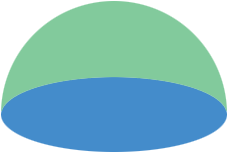SEARCH HOMEMath Central Quandaries & QueriesQuestion from Brenda, a student: What is the surface area of a hemisphere with a radius of 2 inches?Hi Brenda,

The surface area of a sphere of radius $r$ inches is $4 \pi r^2$ square inches where $\pi$ is approximately $3.14159.$ The surface of a hemisphere is composed of two parts, the curved, dome shaped part (green in my diagram) and the flat part (blue in my diagram).The flat part is a circle of radius $r$ inches and it has area $\pi r^2.$ Hence the surface area of the hemisphere is half the surface area of the sphere plus the area of the circular base.

PennyMath Central is supported by the University of Regina and The Pacific Institute for the Mathematical Sciences.